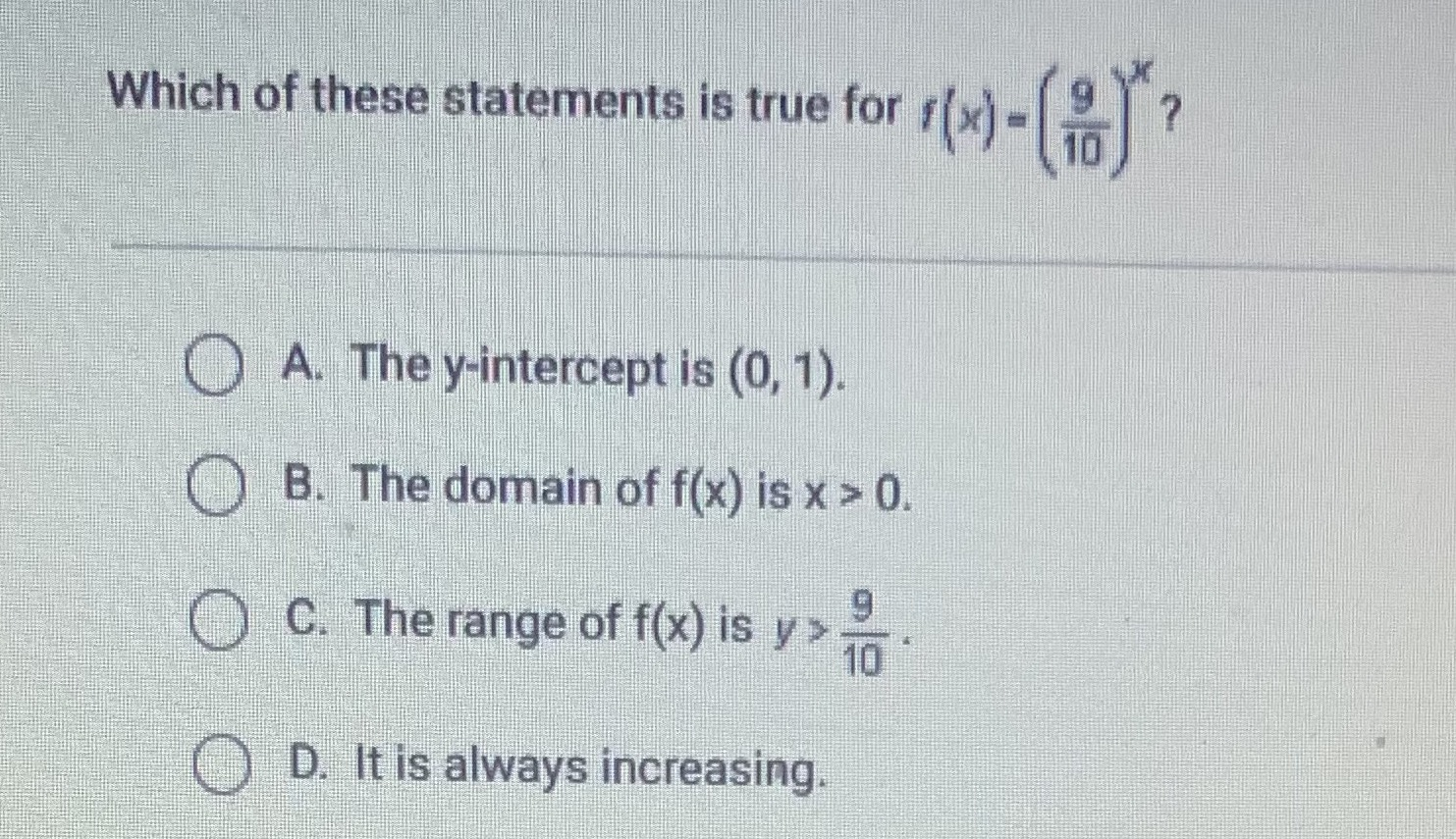### Still have math questions?

Algebra
QuestionWhich of these statements is true for $$f ( x ) = ( \frac { 9 } { 10 } ) ^ { x } ?$$

A. The $$y$$ -intercept is $$( 0,1 )$$ .

B. The domain of $$f ( x )$$ is $$x > 0$$ .

C.The range of $$f ( x )$$ is $$y > \frac { 9 } { 10 }$$ .

D. It is always increasing.